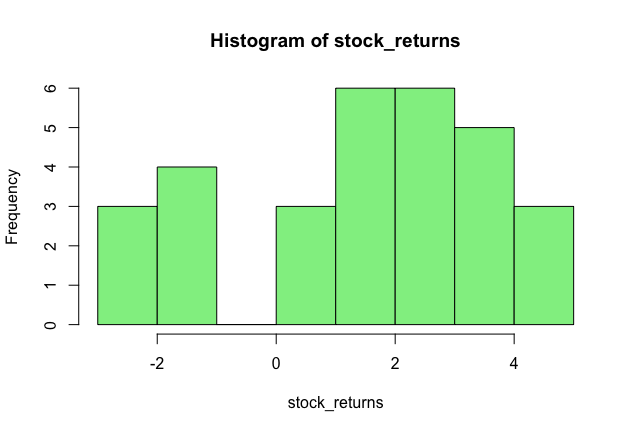# How to Calculate Skewness and Kurtosis in R

Skewness and Kurtosis are two important statistics that tell us about the shape of a distribution. While skewness tells us how skewed a distribution is to the left or right, kurtosis measures the distribution's 'peakedness,' or whether it is heavy-tailed or light-tailed. Learn more about the concepts of skewness and kurtosis.

In this article, we will learn how to calculate the skewness and kurtosis of a dataset in R.

### Dataset

Let’s take the example of the daily stock returns of a stock over the past 30 days.

stock_returns <-  c(4, 3, -1, 2, 4, 5, 3, -2, 1, 5, -3, -1, 4, 5, 3, 3, 2, 4, 2, 1, -2, 4, 3, 2, 2, 1, -1, -1, 2, 3)


### Visualize Data

Before we calculate the skewness and kurtosis, let's visualise the data in the form of a histogram.

hist(stock_returns, col='lightgreen')By looking at the histogram, we can say that the distribution is slightly left skewed, that is, more values are concentrated on the right hand side of the distribution. Also, the distribution seems fat-tailed.

### Calculate Skewness and Kurtosis in R

Let’s verify our observations by calculating the skewness and kurtosis in R. R comes with a library called moments, which contains the functions for skewness and kurtosis. In the following code, we load this library and then calculate the skewness and kurtosis of our dataset.

install.packages('moments')
library(moments)

#calculate skewness
skewness(stock_returns)

 -0.5810439

#calculate kurtosis
kurtosis(stock_returns)

 2.316147


For our stock return data, the skewness is -0.58 and the kurtosis is 2.31. A negative skewness confirms that the data is left-skewed. The kurtosis value is 2.31. The dataset has lighter tails than a normal distribution if the kurtosis is less than 3 (less in the tails).

### Jarque-Bera Test

Using the moments library, we can also perform the Jarque-Bera test using the jarque.test function. This function performs the Jarque-Bera test on the given data sample to determine if the data is drawn from a normal population. Under the hypothesis of normality, data should be symmetrical. Its skewness should be equal to zero and its kurtosis should be close to three.

Let’s perform this test on our data and see what we get.

jarque.test(stock_returns)

Jarque-Bera Normality Test

data:  stock_returns
JB = 2.2726, p-value = 0.321
alternative hypothesis: greater


The p-value is 0.321, which is greater than 0.05. With a p-value >0.05, one would usually say that the data is consistent with having skewness and excess kurtosis of zero. Therefore, we fail to reject the null hypothesis that the dataset has a skewness and kurtosis similar to a normal distribution.